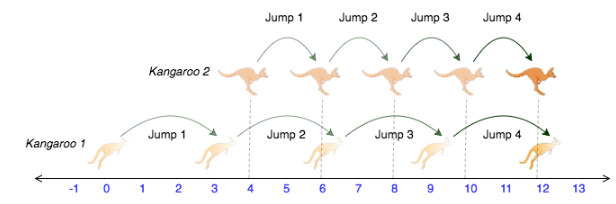# [Python] A program that compares the positions of kangaroos. (while statement)

This is a memo for myself.

▼ Question

――Two kangaroos jump over a certain distance. --Starting points are x1 and x2, respectively. --The jump distances from the second time onward are v1, v2 ――If the two animals come to the same position after several jumps, return "YES". If not, return "NO".URL

▼sample input

#### `python`

``````
x1 = 43
v1 = 2
x2 = 70
v2 = 2
``````

▼sample output

#### `python`

``````
'NO'
``````

#### `python`

``````
def kangaroo(x1, v1, x2, v2):
n = 0
condition = True
while condition:
n += 1
c1 = x1 + v1*n
c2 = x2 + v2*n

#Can't catch up forever
if x1 <= x2 and v1 < v2:
return "NO"
elif x1 < x2 and v1 == v2:
return "NO"
elif x2 <= x1 and v2 > v1:
return "NO"
elif x1 > x2 and v1 == v2:
return "NO"

#May catch up
elif x1 <= x2 and v1 > v2:
if c1 == c2:
return "YES"
elif c1 > c2:
return "NO"
elif x2 <= x1 and v2 > v1:
if c1 == c2:
return "YES"
elif c2 > c1:
return "NO"

if __name__ == '__main__':
fptr = open(os.environ['OUTPUT_PATH'], 'w')

x1V1X2V2 = input().split()

x1 = int(x1V1X2V2)

v1 = int(x1V1X2V2)

x2 = int(x1V1X2V2)

v2 = int(x1V1X2V2)

result = kangaroo(x1, v1, x2, v2)

fptr.write(result + '\n')

fptr.close()
``````

** ・ choreographing ** Choreography You are choreographing a circus show with various animals. Animal choreography circus show

** ・ while **

#### `python`

``````
while conditional expression:
processing
``````

-Repeat the process while the conditional expression is True. ・ Normally, a process that becomes False is inserted. -When ending the return, there is no need to process it as False.